# How to find the optimal number of groups in GBTM

## Background

Group-based trajectory modelling (GBMT) is a way of identifying latent patterns of change from multiple individual trajectories. It is widely used within the field of economics and also becoming popular in health geography. The process of deciding the number of classes is not transparent and tends to be based on one or two model fit statistics. Klijn et al., created a fit-criteria assessment plot (F-CAP) that accepts universal data input to aid the decision on the number of classes (2017).

As we have several data of the macroeconomy, it is important to visually inspect the model fit statistics and to decide the number of classes before applying in a model with the mental health outcomes. These stages (classify and analyse) are normally done seperately, so the units of analysis are classfied, then class membership is taken as known in the subsequent analysis with the health outcome (i.e. deterministic instead of probabilistic).

This document describes the process of using F-CAP’s with the example of trajectories of employment (2006-2015) at the Scottish local authority level (n=32).

## Method

We firstly load the employment data that was processed in the macroeconomy document. Some processing is required to get this data ready for the linear class mixed model. The first figure shows a simple visualisation of the employment trajectories for each LA over the time period:

``````##################### EMPLOYMENT #######################################
library(ggplot2)
library(catspec)
library(gridExtra)
library(lcmm)

# STEP 1: LOAD EMPLOYMENT DATA

# STEP 2 FORMAT DATA FOR LCMM
datamanip<-function(Indicator, scale){
data<-subset(data, IndSub==Indicator)

# Subset for latent class model
traj <- data
traj\$id <- as.factor(traj\$Council)
traj\$x<-traj\$Year
traj\$y<-as.numeric(traj\$Value)
traj<-subset(traj, select=c("id", "x", "y"))
traj\$x2<-traj\$x^2
traj\$x3<-traj\$x^3

# scale by geographic unit!
library(dplyr)
if(scale==TRUE){
traj<-traj %>%
group_by(id) %>%
mutate(y=scale(y, center = TRUE, scale = TRUE))
return(traj)
} else(
return(traj))
}
traj<-datamanip("pct", "FALSE")

# viz
ggplot(traj, aes(as.factor(x), y, group=id)) +
geom_line(aes(colour=id, group=id)) +
labs(x = "Year", y = "% Employed", title = "Plot of all LA trajectories")``````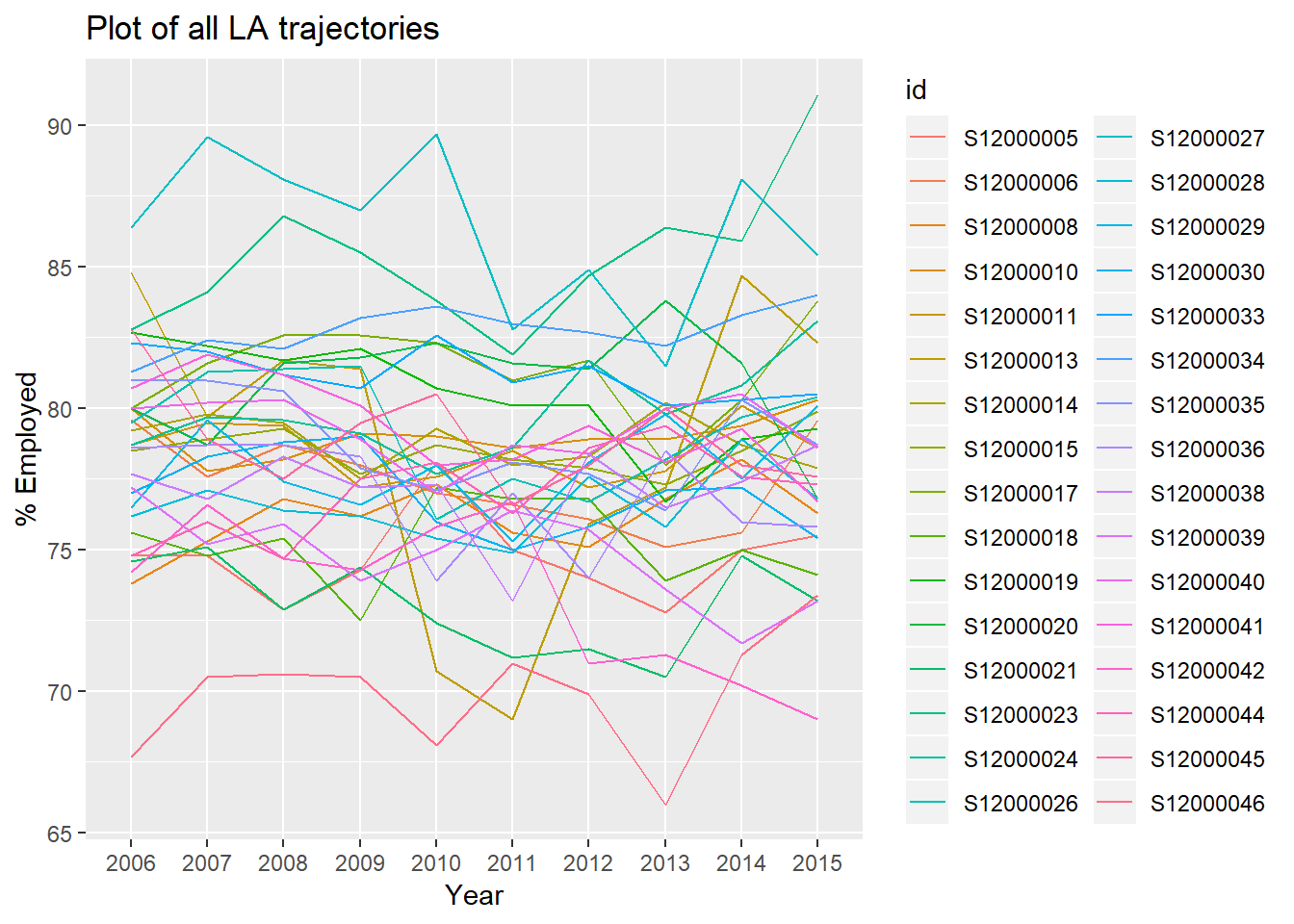Next, we need to fit the LCMM models (this is a batch process taking arguments of ‘formula’, ‘number of groups’ and ‘model’). In this example we are using the linear specification of the employment variables (i.e. we believe that trajectories over time are best modelled by linear terms rather than higher terms), a maximum of 10 latent classes and a linear link (as the employment data is continuous). The model fit statistics are then written into a .csv file that has been formatted to be accepted as universal input by the F-CAP R code.

``````##### STEP 3: FIT THE LCMM MODELS AND OUTPUT MODEL FIT

# Create the file for running the procedure from 2-10 groups
batchit<-function(formula, groups, model){
batch<-expand.grid(formula, rep(2:groups))
colnames(batch)<-c("formula", "numberofgroups")
batch\$formula<-as.character(batch\$formula)
batch\$numberofgroups<-as.numeric(as.character(batch\$numberofgroups))
return(batch)
}
batch<-batchit("x", 10, "linear")

# Model Fit output function
#file.remove(list.files("trajectories/fcap/input/", pattern="Universal_*", full.names = T))
d4<-lcmm(as.formula(paste0("y~", formula)),
as.formula(paste0("mixture=~", formula)),
#as.formula(paste0("random=~", formula)),
# whether to include random effects is quite a talking point
subject='id',
ng=numberofgroups,
idiag=TRUE,
data=traj,
postprob(d4)
conv<-ifelse(d4\$conv==1, "TRUE", "FALSE")
k<-paste(d4\$AIC, d4\$BIC, exp(d4\$loglik), conv)
k<-gsub(" ", ",", k)
pp<-d4\$pprob
pp<-cbind(pp[,3:(ncol(pp))], d4\$pprob\$class)
cat(k, "\n")
# Stop writing to the file
sink()
append=TRUE,
sep = ",",
row.names = F,
col.names = F)
output[is.na(output)]<-""
readr::write_csv(output, paste0("trajectories/fcap/input/","Universal_", numberofgroups,".csv"), col_names = F)
}
# Batch it
library(plyr)
#mdply(batch,modelfittraj)``````

We then take the model fit output and use it as input to the F-CAP function that was supplied by Valeria Lima Passos (co-author of the paper above).

``````##### STEP 4 - Run the CSV files through F-CAP

#source(paste0("trajectories/fcap/fcap_base_12_PC.R"), echo=TRUE)``````

The next step is to look at the F-CAPs and make a decision on the number of classes. Note, where there are + or - signs after some of the model fit statistics, this is the highest and lowest per group, as opposed to the mean. The first plot shows the AIC and BIC (penalises model complexity to a higher degree than AIC). Generally for these two, the lower the number the better the model fit. Six classes has the lowest BIC, but there could be an argument for 4 clases being the elbow.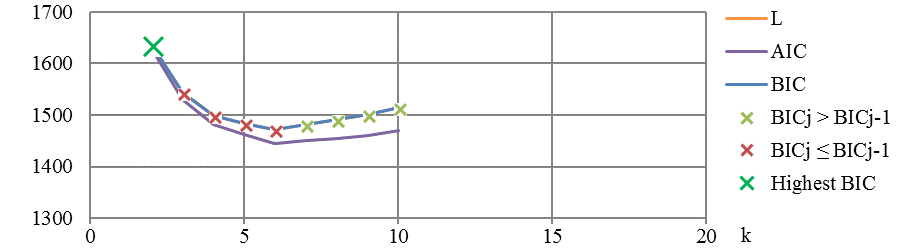The average posterior probability of assignment (APPA) shows the probability of belonging to a certain class. Generally, over 70% for APPA is defined as sufficient. The SD is standard deviation of group membership probabilities and is generally lower when model fit is better. The mismatch between estimated and assigned group probabilities (Mismatch) in a perfect model is zero. In this case, all of the classes have over 70%, with the highest probabilities for 2, then 4 and 5 classes. The mismatch is close to zero for all classes, with slight increasing trend with increasing number of classes. The SD is lowest for 2 groups and is noticebly higher after 5 classes.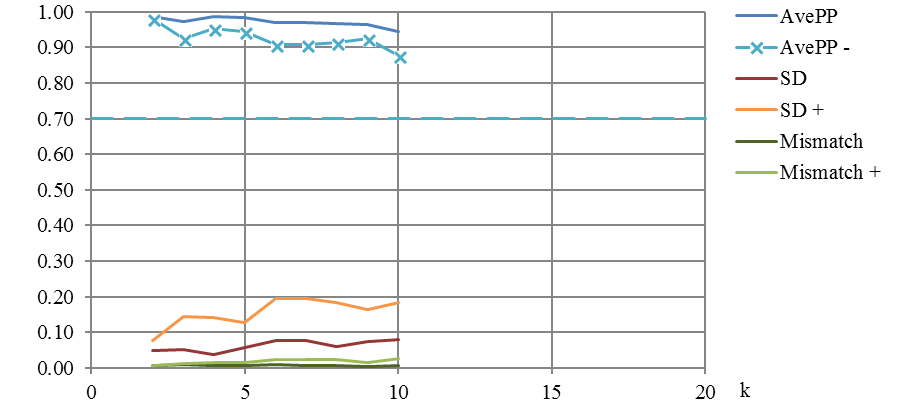The Odds of Correct Classification (OCC) compares the average posterior probability assignment, correcting for OCC based on random assignment. Generally, higher OCC’s indicate better model fit with a threshold of 5 used to show good assignment accuracy. In this case, all classes have sufficient accuracy in class assignment but especially so for classes 3 to 9.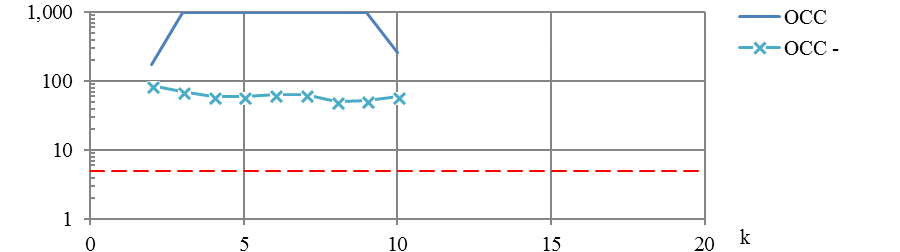We tend not to want classes that contain a very small number of units. A common threshold to apply is that each group will have at least 1% of the sample. However this should be modified if total number of units in the sample are low. In this case, although we are above the 1% threshold in all models with under 6 classes, because we have so few in the sample (N=32), a suitable number of classes would 2,3 or 4, given that at least ~10% of the sample are in each class.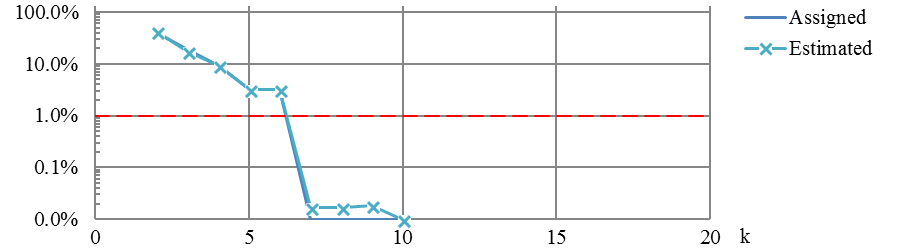In conclusion, the best number of classes in the model of LA employment trajectories from 2006-2015 is four. Although some of the criteria showed little variation between the classes (OCC, Mismatch), we arrived at as this number of classes using the following criteria:

• BIC elbow
• High posterior probabilities (>90%)
• Relatively low standard deviation of PP within each class
• Had at least ~10% of the sample in any one class

The next step is to then see whether changing the polynomial order gives any improvement in these model fit statistics (although in R I can only seem to change all the clusters at once rather than individually). For brevity, this is not shown in this document.

``````## What's the best model?

##### STEP 5 - Finalise the group membership and plot

# plot traj
k<-NULL
traj\$id <- as.factor(traj\$id)
d4<-lcmm(as.formula(paste0("y~", formula)),
as.formula(paste0("mixture=~", formula)),
subject='id',
ng=numberofgroups,
idiag=TRUE,
data=traj,
postprob(d4)
traj\$id2 <- as.numeric(traj\$id)
groups <- as.data.frame(d4\$pprob[,1:2])
traj\$TRAJGROUP <- factor(groups\$class[sapply(traj\$id2, function(x) which(groups\$id==x))])
if (output=="plot"){
ggplot(traj, aes(x, y, group=id, colour=group2)) + geom_line() + geom_smooth(aes(group=group2), method="loess", size=2, se=F) + labs(x="x",y="y",colour="Latent Class")
pl<-ggplot(traj, aes(as.factor(x), y, group=id, colour=TRAJGROUP)) + geom_smooth(aes(group=id, colour=TRAJGROUP),size=0.5, se=F) + geom_smooth(aes(group=TRAJGROUP), method="loess", size=2, se=T) + labs(x="Date",y="% Employed",colour="Latent Class")
pl

} else if (output=="file"){write.csv(traj, paste0("data/",formula, numberofgroups, link,".csv"),row.names=F)}
}

# Put in the F-CAP best model here
plottraj("x", 4, "linear", "plot")``````
``````## Be patient, lcmm is running ...
## The program took 1.2 seconds
##
## Posterior classification:
##   class1 class2 class3 class4
## N   3.00  15.00   3.00  11.00
## %   9.38  46.88   9.38  34.38
##
## Posterior classification table:
##      --> mean of posterior probabilities in each class
##         prob1  prob2  prob3  prob4
## class1 0.9993 0.0007 0.0000 0.0000
## class2 0.0000 0.9976 0.0000 0.0024
## class3 0.0000 0.0000 0.9998 0.0002
## class4 0.0000 0.0476 0.0005 0.9519
##
## Posterior probabilities above a threshold (%):
##          class1 class2 class3 class4
## prob>0.7    100    100    100  90.91
## prob>0.8    100    100    100  90.91
## prob>0.9    100    100    100  90.91
## ``````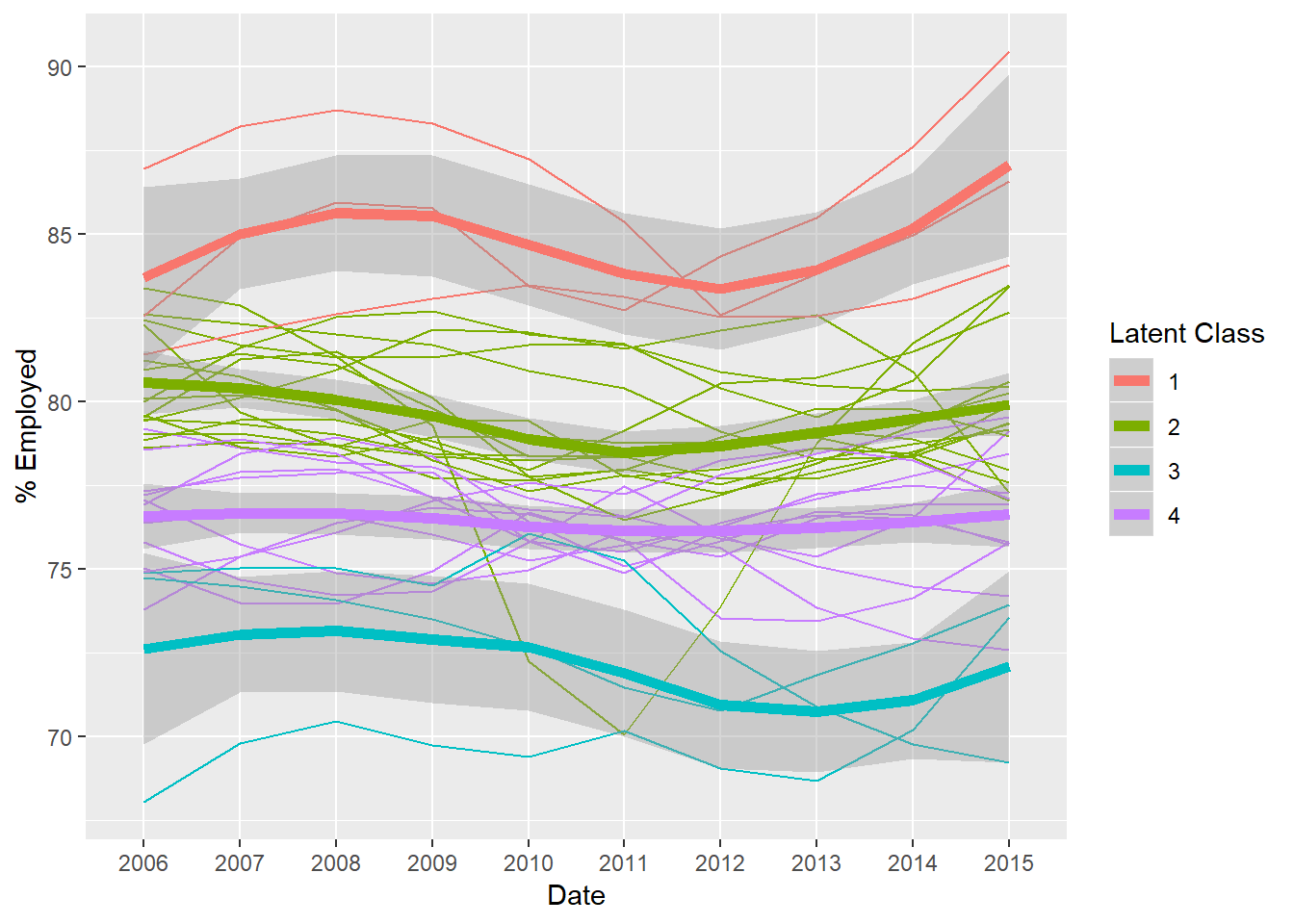The groups are as follows:

• Group 1: Orkney Islands, Shetland Islands, Aberdeenshire
• Group 2: East Lothian, East Renfrewshire, Eilean Siar, Falkirk, Fife, Highland, Midlothian, Moray, Perth and Kinross, Scottish Borders, Aberdeen City, Argyll and Bute , West Lothian, Angus, East Dunbartonshire
• Group 3: North Ayrshire, Dundee City, Glasgow City
• Group 4: Clackmannanshire, Dumfries and Galloway, East Ayrshire, Inverclyde, South Ayrshire, South Lanarkshire, Stirling, City of Edinburgh, Renfrewshire, West Dunbartonshire, North Lanarkshire

n.b We do not include random effects in the above models. These are not included in the the SAS and Stata procedures for GBTM. The implication is that we do not allow variation within or between classes. The practical implication is that this will result in a greater number of latent classes being selected (Further discussion in Saunders, 2011).